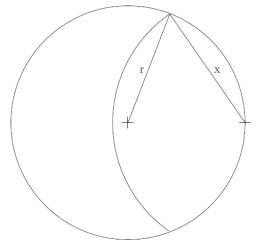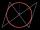# Goat and circles

What is the radius of a circle centered on the other circle and the intersection of the two circles is equal to half the area of the first circle?

This task is the mathematical expression of the role of agriculture. The farmer has circular land on which graze goat. Because the farmer wants to spend the grass throughout the two days, tie it to a stake on the edge of the circle and length of rope that for the first half of grass. Second day give they a whole circle, where they can feed off the remaining one-half of grass.

Correct result:

p=x/r =  116.9 %

#### Solution:

$S_1 + S_2 = \dfrac12 S \ \\ S = \pi r^2 \ \\ S_1 = \dfrac12x^2(\alpha -\sin \alpha) \ \\ S_2 = \dfrac12r^2((2\pi - 2\alpha) -\sin (2\pi - 2\alpha)) \ \\ \ \\ x = 2r \cos (\alpha/2) \ \\ \ \\ \ \\ \alpha = \tan \alpha - \dfrac{ \pi }{ 2 \cos \alpha } \ \\ \ \\ \alpha_1 = 1.9056957293099 = 66^\circ 23'25" \ \\ p_1 = x/r = 1.1587284730181 = 115.87 \% \ \\ \alpha_2 = 4.0903294575997 = -52^\circ 20'34" \ \\ p_2 = x/r = -0.91355336099303 = -91.36 \% \ \\ \alpha_3 = 7.9263876105649 = -78^\circ 2'33" \ \\ p_3 = x/r = -1.3620993158089 = -136.21 \% \ \\ \alpha_4 = 10.756961303274 = 70^\circ 48'29" \ \\ p_4 = x/r = 1.235835638602 = 123.58 \% \ \\ \alpha_5 = -11.0473869356 = 83^\circ 6'1" \ \\ p_5 = x/r = 1.4503719997774 = 145.04 \% \ \\ \alpha_6 = -7.5128894066161 = -93^\circ 36'18" \ \\ p_6 = x/r = -1.6337175982743 = -163.37 \% \ \\ \alpha_7 = -4.8322830401789 = -85^\circ 44'15" \ \\ p_7 = x/r = -1.49640036639 = -149.64 \% \ \\ \ \\ \ \\$We would be pleased if you find an error in the word problem, spelling mistakes, or inaccuracies and send it to us. Thank you!Tips to related online calculators
Do you have a linear equation or system of equations and looking for its solution? Or do you have quadratic equation?
Most natural application of trigonometry and trigonometric functions is a calculation of the triangles. Common and less common calculations of different types of triangles offers our triangle calculator. Word trigonometry comes from Greek and literally means triangle calculation.

#### You need to know the following knowledge to solve this word math problem:

We encourage you to watch this tutorial video on this math problem:

## Next similar math problems:

• Isosceles trapezoidFind the area of an isosceles trapezoid with bases of 8cm and 72mm. The height of the trapezoid is equal to three-quarters of the longer base.
• Area of RTCalculate the area of a right triangle that hypotenuse has length 14, and one hypotenuse segment has length 5.
• Square gardensThe gardening colony with dimensions of 180 m and 300 m is to be completely divided into equally large square areas with the largest possible area. Calculate how many such square areas can be obtained and determine the side length of the square.
• Isosceles triangleCalculate the area of an isosceles triangle, the base of which measures 16 cm and the arms 10 cm.
• Circle in rhombusIn the rhombus is inscribed circle. Contact points of touch divide the sides to parts of length 19 cm and 6 cm. Calculate the circle area.
• GardensThe area of the square garden is 3/4 of the area of the triangular garden with sides of 80 m, 50 m, 50 m. How many meters of the fence do we need to fence a square garden?
• Sides of the triangleCalculate triangle sides where its area is S = 84 cm2 and a = x, b = x + 1, xc = x + 2
• Euklid4Legs of a right triangle have dimensions 244 m and 246 m. Calculate the length of the hypotenuse and the height of this right triangle.
• Without Euclid lawsRight triangle ABC with right angle at the C has a=5 and hypotenuse c=19. Calculate the height h of this triangle without the use of Euclidean laws.
• Right ΔA right triangle has the length of one leg 11 cm and length of the hypotenuse 61 cm. Calculate the height of the triangle.
• Area and perimeter of rectangleThe content area of the rectangle is 3000 cm2, one dimension is 10 cm larger than the other. Determine the perimeter of the rectangle.
• Squares above sidesTwo squares are constructed on two sides of the ABC triangle. The square area above the BC side is 25 cm2. The height vc to the side AB is 3 cm long. The heel P of height vc divides the AB side in a 2: 1 ratio. The AC side is longer than the BC side. Calc
• Hypotenuse - RTA triangle has a hypotenuse of 55 and an altitude to the hypotenuse of 33. What is the area of the triangle?
• Railway embankmentThe section of the railway embankment is an isosceles trapezoid, the sizes of the bases of which are in the ratio 5: 3. The arms have a length of 5 m and the height of the embankment is 4.8 m. Calculates the size of the embankment section area.
• Right isosceles triangleRight isosceles triangle has an altitude x drawn from the right angle to the hypotenuse dividing it into 2 equal segments. The length of one segment is 5 cm. What is the area of the triangle?
• Free space in the gardenThe grandfather's free space in the garden was in the shape of a rectangular triangle with 5 meters and 12 meters in length. He decided to divide it into two parts and the height of the hypotenuse. For the smaller part creates a rock garden, for the large
• Isosceles triangle 9Given an isosceles triangle ABC where AB= AC. The perimeter is 64cm and altitude is 24cm. Find the area of the isosceles triangle